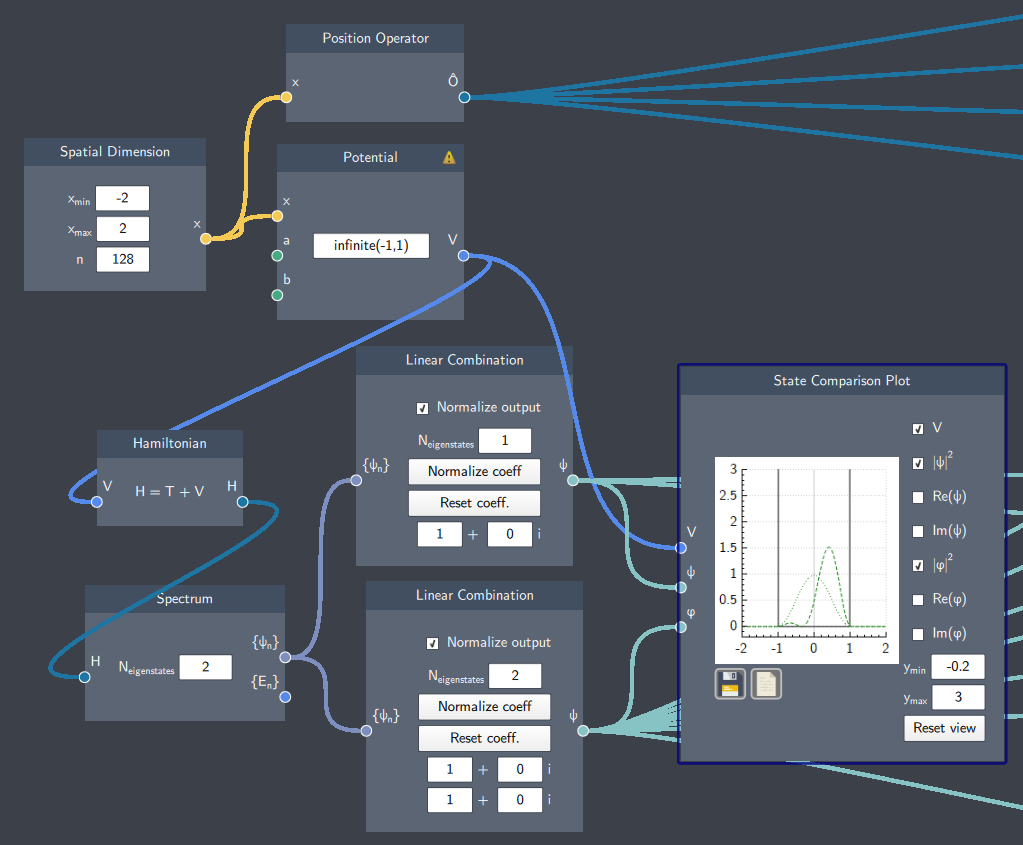# State AnalysisThe overlap
Calculates the overlap $\langle \psi_1 | \psi_2 \rangle$ between two states.Expectation value

Calculate the expectation-value of an arbitrary Operator with regard to an arbitrary state.

for input $\hat{O}$ and $\psi$ calculates $\langle \psi | \hat{O} | \psi \rangle$Squared expectation value

Calculate the squared expectation-value of an arbitrary Operator with regard to an arbitrary state.

for input $\hat{O}$ and $\psi$ calculates  $\langle \psi | \hat{O}^2 | \psi \rangle$.Standard deviation.
Calculate the standard deviation $\sigma = \sqrt{\langle\hat{O}^2 \rangle - \langle\hat{O} \rangle ^2}$ of an operator $\hat{O}$.Varience
Calculate the variance $\sigma^2= \langle \hat{O}^2 \rangle- \langle \hat{O} \rangle ^2$ of an operator $\hat{O}$.Fidelity
Calculate the fidelity $F = |\langle \psi | \phi \rangle |^2$ between two statesIntegration
Calculates the integral $\int_a^b |\psi|^2 dx$ of the norm squared of a state

In this section the nodes for the state analysis is presented. In order to do so a simple system with the infinite well as a potential is used along with the ground state $\psi_g(x) = \psi_1(x)$ and the superposition $\psi_s(x) = \psi_1(x) + \psi_2(x)$.We investigate the

• The expectation value $\langle \hat{O} \rangle$
• The squared expectation value $\langle \hat{O}^2 \rangle$
• The standard deviation $\sigma$
• The variance $\sigma^2$

with the position operator on the superposition state $\psi_s$.

The overlap between the two states $\psi_g$ and $\psi_s$  can be found using the node $\langle \psi | \psi \rangle$.
The fidelity between the two states can be found using the fidelity node $| \langle \psi | \phi \rangle |^2$.
If you would like to investigate a portion of the distribution in an interval use the integration node. $\int |\psi|^2$